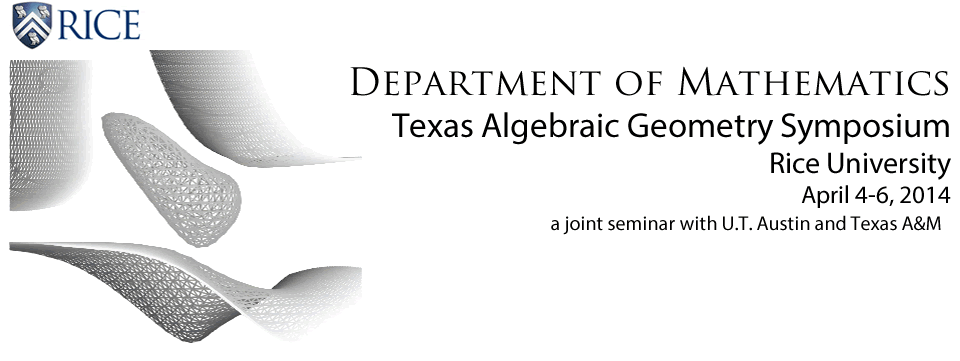## Texas Algebraic Geometry Conference Abstracts

Rational curves on holomorphic symplectic varieties, Brendan Hassett (Rice University)

Holomorphic symplectic manifolds are higher-dimensional generalizations of K3 surfaces. We will survey results on the structure of rational curves on these varieties.

Between Characteristic 0 and Characteristic p, Jacob Lurie (Harvard University)

In classical algebraic geometry, there is often a stark difference between the behavior of algebraic varieties over fields of characteristic zero (such as the complex numbers) and algebraic varieties over fields of characteristic p (such as finite fields). In the setting of spectral algebraic geometry, one can consider an entire family of "regimes" which in some sense interpolate between characteristic zero and characteristic p. In this talk, I will give an informal introduction to the language of spectral algebraic geometry and then discuss some curious behavior of "roots of unity" in these intermediate regimes.

Actions of correspondences and local terms, Martin Olsson (University of California, Berkeley)

In this talk I will give an overview of a continuing project aimed at understanding motivic aspects of correspondences acting on $\ell$-adic sheaves. This work is motivated by trying to prove various independence of $\ell$ results, but also leads to several interesting problems in intersection theory which I will highlight.

Uniform boundedness of the Brauer group, R. Parimala (Emory University)

Let F be a field and Br(F) the Brauer group of F. We say that the p-torsion of Br(F) is uniformly bounded if there is an integer Np such that given finitely many elements in the p-torsion of Br(F), there is an extension L of F of degree at most Np which splits all these elements. Number fields are examples of fields with uniform boundedness property for the Brauer group. We shall explain how uniform boundedness for the 2-torsion of Br(F) leads to interesting consequences concerning zeros of quadratic forms. We also give a class of examples of fields with uniform boundedness property. (joint work with V. Suresh)

Boundary components of Mumford-Tate domains, Gregory Pearlstein (Texas A&M University)

Mumford-Tate groups arise as the natural symmetry groups of Hodge structures and their variations. I describe recent work with Matt Kerr on computing the Mumford-Tate group of the Kato-Usui boundary components of a degeneration of Hodge structure (talk outline).

F-singularities in families, Karl Schwede (Penn State University)

F-singularities are classes of singularities defined by the behavior Frobenius. A prominent tool for measuing these singularities is the test ideal, a characteristic p > 0 analog of the multiplier ideal. Recently, there has been interest in applying the methods of F-singularities to a number of geometric problems in positive characteristic. However, one gap in the theory has been the behavior of F-singularities in families. For example restriction theorems for test ideals have been lacking. In this talk, I will discuss recent joint work with Zsolt Patakfalvi and Wenliang Zhang on the behavior of F-singularities and test ideals in families. For example, we will obtain generic (and non-generic) restrictions theorems for test ideals. Some global geometric consequences will also be discussed if there is sufficient time.

The Büchi K3 surface and its rational points, Damiano Testa (University of Warwick)

In order to extend Matiyasevich's resolution of Hilbert's Tenth Problem, Büchi introduced a sequence of affine algebraic surfaces: he showed that if the surfaces in this sequence eventually only have trivial integral solutions, then the proof of undecidability can be extended to the case of systems of diagonal quadratic equations. Vojta later showed that a weak form of the Lang's Conjectures implies that, with finitely many exceptions, the "Büchi surfaces" do indeed only have trivial integral solutions.

In my talk I will report on joint work in progress with M. Artebani and A. Laface on the rational (not necessarily integral!) points of the first non-rational surface in Büchi's sequence. I will mention some of the geometric properties of this surface and show that it is a moduli space of vector bundles. The modular interpretation of this problem naturally leads to a question on integral structures on a moduli spaces of vector bundles, to which we do not know the answer.raic constructions arising in the framework of anabelian geometry of higher-dimensional function fields (joint with F. Bogomolov).

Basic field theory, Yuri Tschinkel (NYU/Simons Foundation)

I will describe some geometric and algebraic constructions arising in the framework of anabelian geometry of higher-dimensional function fields (joint with F. Bogomolov).

Organizing committee:
Brendan Hassett